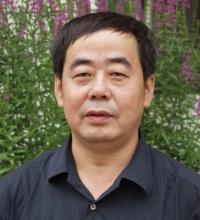Local Discontinuous Galerkin Method Coupled with Implicit Integration Factor Methods for Reaction-Diffusion Systems
Speaker
Prof. Xi-Jun Yu
Institute of Applied Physics and Computational Mathematics
Abstract

In this talk, on two-dimension unstructured triangular meshes, an efficient fully -discrete local discontinuous Galerkin (LDG) method is presented for solving the nonlinear reaction-diffusion systems, which are often used as mathematical models for many biological, physical and chemical sciences. In order to overcome LDG method for the strict time-step restriction Δt = O(h²min) of explicit schemes for stability, the implicit integration factor (IIF) method based on Krylov subspace approximation is introduced into the temporal discretization, in which the time-step can be taken as Δt = O(hmin) . The method can derive the numerical approximations not only for solutions but also for their gradients at the same time comparing other methods for the numerical solutions only. Moreover, the method can compute element by element and avoid solving a global system of nonlinear algebraic equations as the standard implicit schemes do, so that the method can reduce the computational cost greatly. Numerical experiments for the reaction-diffusion equations with exact solutions and the morphogenesis systems from developmental biology are conducted to illustrate the efficient, accurate and advantages of the LDG method coupled with the Krylov IIF time discretization.